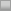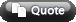View Single Post09-10-2009, 12:56 PM #2 jason1990 old hand   Join Date: Sep 2004 Posts: 1,876 Re: How to include LaTeX images in your posts The following post supersedes the original post of this thread. In the following post, a typo was corrected and some formatting was modified. -------------------- To post LaTeX formulas as images in your posts, use the following syntax: [img]http://latex.codecogs.com/gif.latex?insert LaTeX code here[/img]For example, the LaTeX image: is produced by the syntax: [img]http://latex.codecogs.com/gif.latex?\Phi(x) = \frac1{\sqrt{2\pi}} \int_{-\infty}^x e^{-u^2/2} \, du[/img]Here are some helpful hints to remember: It is okay to include spaces in your LaTeX code, but do not use carriage returns. For example, before posting the following LaTeX code: f(x) = \begin{cases} x^2 &\text{if $x \ge 0$},\\ 0 &\text{if $x < 0$}. \end{cases}the carriage returns must be removed. The required syntax is: [img]http://latex.codecogs.com/gif.latex? f(x) = \begin{cases} x^2 &\text{if $x \ge 0$},\\ 0 &\text{if $x < 0$}. \end{cases} [/img]which produces the following image: Some characters that are used in LaTeX code are not recognized by the forum software. These characters must be replaced by their URL Escape Codes. (A list of these codes can be found here: Percent-encoding on Wikipedia.) At the time of this writing, the only character we have found that requires a URL Escape Code is the asterisk, "*". Its URL Escape Code is %2A. For instance, the LaTeX image: must be produced by the syntax: [img]http://latex.codecogs.com/gif.latex?\Psi = \Phi\Phi^%2A[/img] If you cannot see these LaTeX images, make sure that you have the right settings selected. Go to My 2+2 --> Edit Options, and make sure that "Show Images (including attached images and images in [IMG] code)" is checked. If you are new to LaTeX, you may want to see the LaTeX guide on Wikibooks. The Mathematics and Advanced Mathematics sections may be particularly useful. Last edited by Zeno; 09-10-2009 at 11:32 PM.